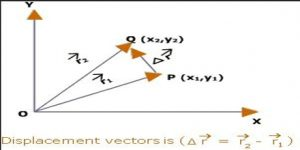# Displacement Vector

Displacement Vector: Distance traveled by a point along a linear or straight path is called the displacement vector. It is a vector which gives the position of a point with reference to a point other than the origin of the coordinate system.

It is represented by Ř.

Explanation: Suppose, the distance traveled by a point along a straight path is, AB = R.

So, R is the displacement vector.Displacement=ΔR = R2 – R1

​R2 refers to the value of the final position.

R1 refers to the value of the initial position.

ΔR is the symbol used to represent displacement.